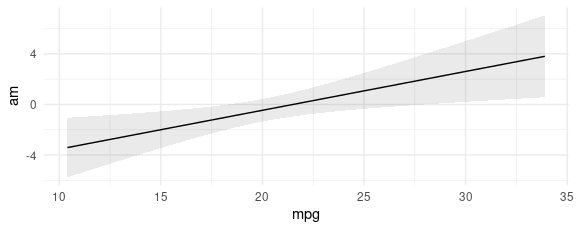In the context of this package, an “Adjusted Prediction” is defined as:

The response predicted by a model for some combination of the regressors’ values, such as their means or factor levels (a.k.a. “reference grid”).

An adjusted prediction is thus the regression-adjusted response variable (or link, or other fitted value), for a given combination (or grid) of predictors. This grid may or may not correspond to the actual observations in a dataset.

By default, predictions calculates the regression-adjusted predicted values for every observation in the original dataset:

library(marginaleffects)

mod <- lm(mpg ~ hp + factor(cyl), data = mtcars)

pred <- predictions(mod)

#>       type predicted std.error conf.low conf.high  mpg  hp cyl
#> 1 response  20.03819 1.2041405 17.57162  22.50476 21.0 110   6
#> 2 response  20.03819 1.2041405 17.57162  22.50476 21.0 110   6
#> 3 response  26.41451 0.9619738 24.44399  28.38502 22.8  93   4
#> 4 response  20.03819 1.2041405 17.57162  22.50476 21.4 110   6
#> 5 response  15.92247 0.9924560 13.88952  17.95543 18.7 175   8
#> 6 response  20.15839 1.2186288 17.66214  22.65463 18.1 105   6

In many cases, this is too limiting, and researchers will want to specify a grid of “typical” values over which to compute adjusted predictions.

Predictions over a “typical” data grid

There are two main ways to select the reference grid over which we want to compute adjusted predictions. The first is using the variables argument. The second is with the newdata argument and the datagrid() function that we already introduced in the marginal effects vignette.

variables: Levels and Tukey’s 5 numbers

The variables argument is a handy shortcut to create grids of predictors. Each of the levels of factor/logical/character variables listed in the variables argument will be displayed. For numeric variables, predictions will compute adjusted predictions at Tukey’s 5 summary numbers. All other variables will be set at their means or modes.

The data.frame produced by predictions is “tidy”, which makes it easy to manipulate with other R packages and functions:

cyl
hp 4 6 8
52 27.40010 21.43244 18.87925
96 26.34239 20.37474 17.82154
123 25.69334 19.72569 17.17249
180 24.32313 18.35547 15.80228
335 20.59711 14.62945 12.07626

newdata and datagrid

A second strategy to construct grids of predictors for adjusted predictions is to combine the newdata argument and the datagrid function. Recall that this function creates a “typical” dataset with all variables at their means or modes, except those we explicitly define:

We can also use this datagrid function in a predictions call (omitting the model argument):

Users can change the summary function used to summarize each type of variables using the FUN.numeric, FUN.factor, and related arguments, for example substituting the mean for the median.

counterfactual data grid

An alternative approach to construct grids of predictors is to use grid.type = "counterfactual" argument value. This will duplicate the whole dataset, with the different values specified by the user.

For example, the mtcars dataset has 32 rows. This command produces a new dataset with 64 rows, with each row of the original dataset duplicated with the two values of the am variable supplied (0 and 1):

Then, we can use this dataset and the predictions function to create interesting visualizations: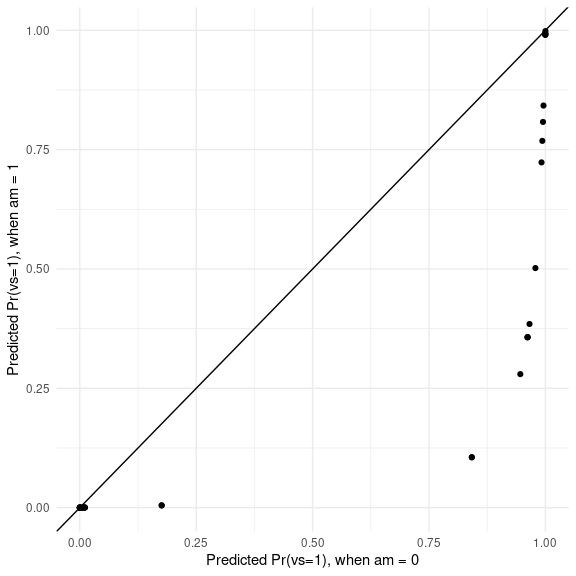In this graph, each dot represents the predicted probability that vs=1 for one observation of the dataset, in the counterfactual worlds where am is either 0 or 1.

Plot: Conditional Predictions

First, we download the ggplot2movies dataset from the RDatasets archive. Then, we create a variable called certified_fresh for movies with a rating of at least 8. Finally, we discard some outliers and fit a logistic regression model:

library(tidyverse)
mutate(style = case_when(Action == 1 ~ "Action",
Comedy == 1 ~ "Comedy",
Drama == 1 ~ "Drama",
TRUE ~ "Other"),
style = factor(style),
certified_fresh = rating >= 8) %>%
filter(length < 240)

mod <- glm(certified_fresh ~ length * style, data = dat, family = binomial)

We can plot adjusted predictions, conditional on the length variable using the plot_cap function:

mod <- glm(certified_fresh ~ length, data = dat, family = binomial)

plot_cap(mod, condition = "length")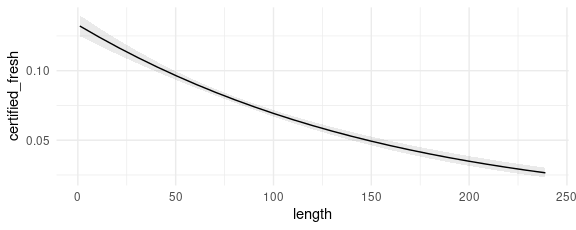We can also introduce another condition which will display a categorical variable like style in different colors. This can be useful in models with interactions:

mod <- glm(certified_fresh ~ length * style, data = dat, family = binomial)

plot_cap(mod, condition = c("length", "style"))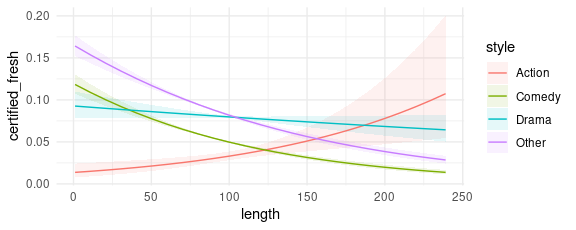Of course, you can also design your own plots or tables by working with the predictions output directly:

predictions(mod,
newdata = datagrid(length = 90:120,
style = c("Action", "Comedy"))) %>%
ggplot(aes(length, predicted, color = style))  +
geom_line() +
facet_wrap(~type, scales = "free_y")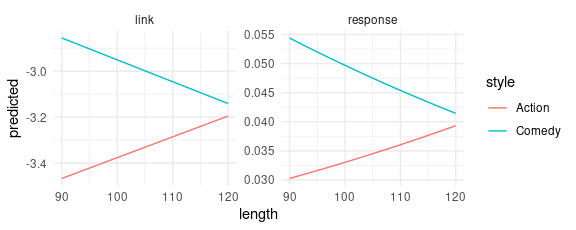Prediction types

The predictions function computes model-adjusted means on the scale of the output of the predict(model) function. By default, predict produces predictions on the "response" scale, so the adjusted predictions should be interpreted on that scale. However, users can pass a string or a vector of strings to the type argument, and predictions will consider different outcomes.

Typical values include "response" and "link", but users should refer to the documentation of the predict of the package they used to fit the model to know what values are allowable. documentation.

mod <- glm(am ~ mpg, family = binomial, data = mtcars)
pred <- predictions(mod, type = c("response", "link"))
#>       type predicted  std.error  conf.low conf.high am  mpg
#> 1 response 0.4610951 0.11584004 0.2554723 0.6808686  1 21.0
#> 2 response 0.4610951 0.11584004 0.2554723 0.6808686  1 21.0
#> 3 response 0.5978984 0.13239819 0.3356711 0.8139794  1 22.8
#> 4 response 0.4917199 0.11961263 0.2746560 0.7119512  0 21.4
#> 5 response 0.2969009 0.10051954 0.1411369 0.5204086  0 18.7
#> 6 response 0.2599331 0.09782666 0.1147580 0.4876032  0 18.1

Users who need more control over the type of adjusted predictions to compute, including a host of options for back-transformation, may want to consider the emmeans package.

We can also plot predictions on different outcome scales:

plot_cap(mod, condition = "mpg", type = "response")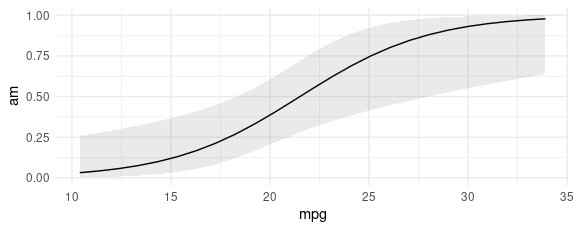plot_cap(mod, condition = "mpg", type = "link")